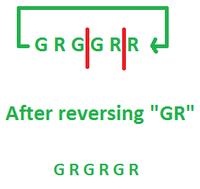Open in App
Not now

# Make the consecutive characters distinct by using given operation

• Last Updated : 01 Feb, 2023

Given a cyclic connected String S (Cyclic string means start and end are connected with each other) containing characters only ‘R’ and ‘G’. the task is to return YES or NO if it is possible to make all the consecutive characters different or not by making 2 cuts in the S, which will divide the string into two pieces, then reverse one of these pieces and tie the endpoints of both strings.

Examples:

Input: S = “RGRGRG”
Output: YES
Explanation: In the given string all the adjacent characters are already different

Input: S = “GRGGRR”
Output: YES
Explanation: The operation is performed as:

• Make two cuts between index 3, 4 and 5, 6, So the S divides into two parts:Graphical explanation of test case 2

• Reverse the “GR” piece of the string and merge it into the same place.

Now it can be verified that the string has all possible adjacent characters that are different including the first and last characters, They are also adjacent.

Approach: Implement the idea below to solve the problem:

If there are more than 2 pairs of the same adjacent characters or the count of R and G characters is different then the answer is NO else answer is YES

Steps were taken to solve the problem:

• Declare an integer N and initialize it equal to the S.length(), for holding String’s length.
• Declare and initialize two integers G and R to 0.
• Run a loop from i=0 to less than N and follow the below-mentioned steps:
• If ( S.charAt(i) == S.charAt((i+1)) )
• If(S.charAt(i) == ‘G’) then G++ else R++
• if ((g == 1 && r == 1) || (g == 0 && r == 0)) then output YES else NO.

Below is the code to implement the approach:

## C++

 `#include ` `using` `namespace` `std;`   `void` `Is_Possible(string str)` `{`   `  ``int` `n = str.length();` `  ``int` `g = 0, r = 0;`   `  ``for` `(``int` `i = 0; i < n; i++) {`   `    ``// If adjacent characters` `    ``// are equal` `    ``if` `(str[i] == str[(i + 1) % n]) {`   `      ``if` `(str[i] == ``'G'``)` `        ``g++;` `      ``else` `        ``r++;` `    ``}` `  ``}`   `  ``if` `((g == 1 && r == 1) || (g == 0 && r == 0))` `    ``cout<<``"YES"``;` `  ``else` `    ``cout<<``"NO"``;` `}`   `int` `main()` `{`   `  ``// Input String` `  ``string str = ``"GRRRGGR"``;`   `  ``// Function call` `  ``Is_Possible(str);` `  ``return` `0;` `}`   `// This code is contributed by poojaagrawal2.`

## Java

 `// Java code to implement the approach`   `import` `java.io.*;` `import` `java.lang.*;` `import` `java.util.*;`   `class` `GFG {`   `    ``// Driver Function` `    ``public` `static` `void` `main(String[] args)` `        ``throws` `java.lang.Exception` `    ``{`   `        ``// Input String` `        ``String str = ``"GRRRGGR"``;`   `        ``// Function call` `        ``Is_Possible(str);` `    ``}`   `    ``// Method for checking if we can` `    ``// make adjacents different or not` `    ``static` `void` `Is_Possible(String str)` `    ``{`   `        ``int` `n = str.length();` `        ``int` `g = ``0``, r = ``0``;`   `        ``for` `(``int` `i = ``0``; i < n; i++) {`   `            ``// If adjacent characters` `            ``// are equal` `            ``if` `(str.charAt(i) == str.charAt((i + ``1``) % n)) {`   `                ``if` `(str.charAt(i) == ``'G'``)` `                    ``g++;` `                ``else` `                    ``r++;` `            ``}` `        ``}`   `        ``if` `((g == ``1` `&& r == ``1``) || (g == ``0` `&& r == ``0``))` `            ``System.out.println(``"YES"``);` `        ``else` `            ``System.out.println(``"NO"``);` `    ``}` `}`

## Python3

 `# Python code to implement the approach`   `def` `Is_Possible(``str``):` `    ``n``=``len``(``str``)` `    ``g,r``=``0``,``0` `    `  `    ``for` `i ``in` `range``(n):` `        ``# If adjacent characters` `        ``# are equal` `        ``if` `(``str``[i] ``=``=` `str``[(i ``+` `1``) ``%` `n]):` `            ``if` `(``str``[i] ``=``=` `'G'``):` `                ``g``+``=``1` `            ``else``:` `                ``r``+``=``1` `    ``if` `((g ``=``=` `1` `and` `r ``=``=` `1``) ``or` `(g ``=``=` `0` `and` `r ``=``=` `0``)):` `        ``print``(``"YES"``)` `    ``else``:` `        ``print``(``"NO"``)`   `# Input String` `str` `=` `"GRRRGGR"`   `# Function call` `Is_Possible(``str``)`   `# This code is contributed by Pushpesh Raj.`

## C#

 `// C# implementation of the above approach`   `using` `System;` `using` `System.Collections.Generic;`   `class` `GFG {`   `  ``static` `void` `Is_Possible(``string` `str)` `  ``{`   `    ``int` `n = str.Length;` `    ``int` `g = 0, r = 0;`   `    ``for` `(``int` `i = 0; i < n; i++) {`   `      ``// If adjacent characters` `      ``// are equal` `      ``if` `(str[i] == str[(i + 1) % n]) {`   `        ``if` `(str[i] == ``'G'``)` `          ``g++;` `        ``else` `          ``r++;` `      ``}` `    ``}`   `    ``if` `((g == 1 && r == 1) || (g == 0 && r == 0))` `      ``Console.Write(``"YES"``);` `    ``else` `      ``Console.Write(``"NO"``);` `  ``}`   `  ``public` `static` `void` `Main()` `  ``{`   `    ``// Input String` `    ``string` `str = ``"GRRRGGR"``;`   `    ``// Function call` `    ``Is_Possible(str);` `  ``}` `}`   `// This code is contributed by poojaagrawal976.`

## Javascript

 `// Javascript code to implement the approach`   `// Method for checking if we can` `// make adjacents different or not` `function` `Is_Possible(str)` `{`   `    ``let n = str.length;` `    ``let g = 0, r = 0;`   `    ``for` `(let i = 0; i < n; i++) {`   `        ``// If adjacent characters` `        ``// are equal` `        ``if` `(str[i] == str[(i+1)%n]) {`   `            ``if` `(str[i] == ``'G'``)` `                ``g++;` `            ``else` `                ``r++;` `        ``}` `    ``}`   `    ``if` `((g == 1 && r == 1) || (g == 0 && r == 0))` `        ``console.log(``"YES"``);` `    ``else` `        ``console.log(``"NO"``);` `}`   `// Driver Function`   `// Input String` `let str = ``"GRRRGGR"``;`   `// Function call` `Is_Possible(str);`   `// This code is contributed by ratiagarawal.`

Output

`NO`

Time Complexity: O(N)
Auxiliary Space : O(1)

Related Articles:

My Personal Notes arrow_drop_up
Related Articles